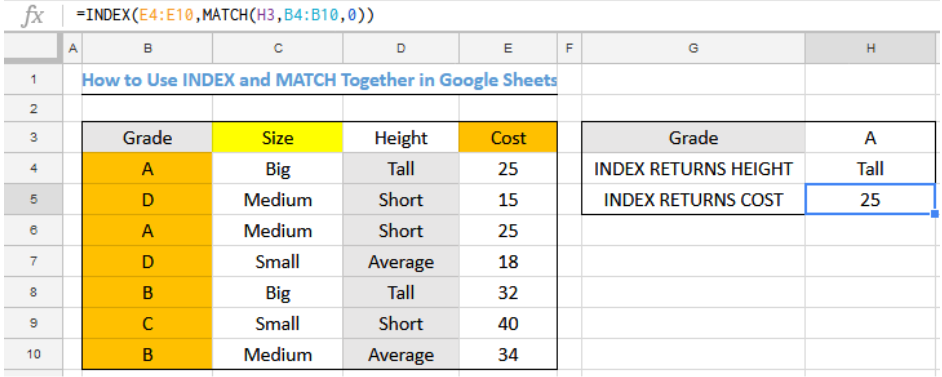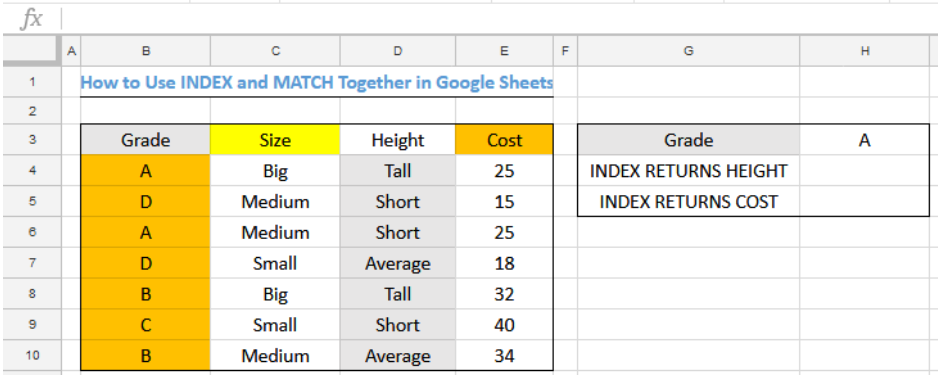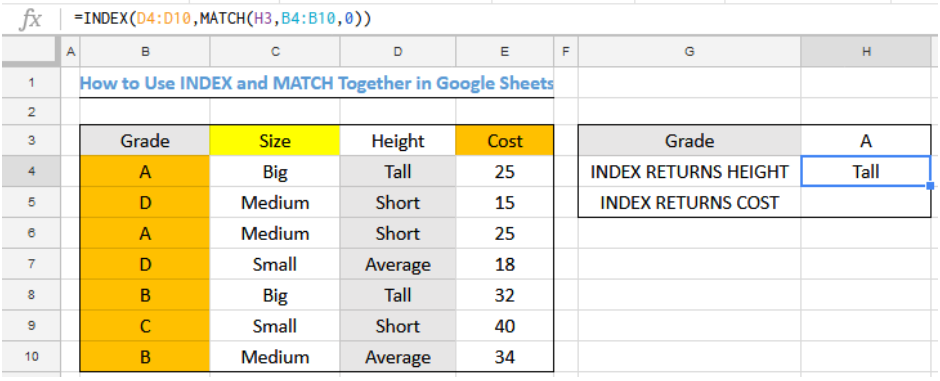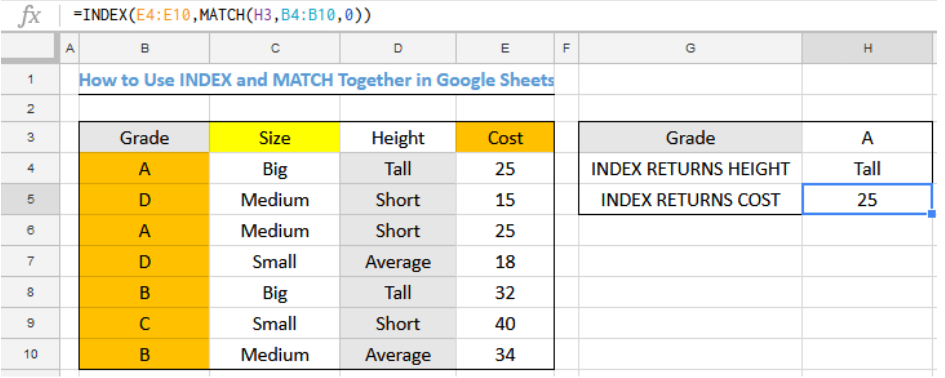Get instant live expert help with Excel or Google Sheets“My Excelchat expert helped me in less than 20 minutes, saving me what would have been 5 hours of work!”

#### Post your problem and you’ll get Expert help in seconds.

Your message must be at least 40 characters
Our professional Expert are available now. Your privacy is guaranteed.

# How to Use INDEX and MATCH Together in Google Sheets

We can use a formula that is based on the INDEX and MATCH functions to lookup a value in a table in a Google Sheet. Unlike in excel where the result is entered with CRTL+SHIFT+ENTER, the result is entered with the enter key only for Google Sheets. The steps below will walk through the process.Figure 1- How to Use INDEX and MATCH Together in Google Sheets

## General Formula

`=INDEX(range1,MATCH(A1,range2),0))`

• Range1 is the array of values from which INDEX returns the final result
• Range2 is the array of values that help INDEX to identify the position of the value to return

## Formula

INDEX RETURNS HEIGHT:` =INDEX(D4:D10,MATCH(H3,B4:B10,0))`

INDEX RETURNS COST: `=INDEX(E4:E10,MATCH(H3,B4:B10,0))`

## Setting up the Data

We will use the INDEX and MATCH functions to get the Height and the Cost of the A grade item in figure 2. We must note that where there are similar values that could be returned, INDEX returns the first matching value.Figure 2 – Setting up the Data

## Lookup HEIGHT with INDEX and MATCH Functions

• We will click on Cell H4
• We will insert the formula below into Cell H4
`=INDEX(D4:D10,MATCH(H3,B4:B10,0))`
• We will press ENTERFigure 3- Result for Lookup of Height with INDEX and MATCH functions

## Lookup COST with INDEX and MATCH Functions

• We will click on Cell H5
• We will insert the formula below into Cell H5
`=INDEX(E4:E10,MATCH(H3,B4:B10,0))`
• We will press ENTERFigure 4- Result for Lookup of Cost with INDEX and MATCH functions

## Explanation

INDEX RETURNS HEIGHT:` =INDEX(D4:D10,MATCH(H3,B4:B10,0))`

In this formula, the MATCH function looks for the value of Cell H3 in Range B4:B10. 0 signifies that we require an exact match. The MATCH FUNCTION returns the position 1 and 3. Because INDEX returns the first matching value, TALL is returned as the height.

## Instant Connection to an Expert through our Excelchat Service

Most of the time, the problem you will need to solve will be more complex than a simple application of a formula or function. If you want to save hours of research and frustration, try our live Excelchat service! Our Excel Experts are available 24/7 to answer any Excel question you may have. We guarantee a connection within 30 seconds and a customized solution within 20 minutes.